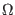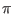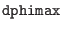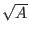Next: TJM Model Temperature Dependence Up: Josephson Junction Model Previous: RSJ Model Temperature Dependence   Contents   Index

### Josephson Tunnel Junction Model

For level=3, a microscopic tunnel junction "Werthamer" model, also known as a tunnel junction model (TJM) is indicated. The model is more physics-based that the empirical RSJ model. The formulation follows the method of

A. A. Odintsov, V. K. Semenov and A. B. Zorin, IEEE Trans. Magn. 23, 763 (1987).
as implemented in the the open-source MitMoJCo project on https://github.com/drgulevich/mitmojco. The actual model computations make use of predefined fitting parameters that can be produced with the mmjco program provided with WRspice (B.1). The mmjco program integrates the tunneling current expressions producing a tunnel current amplitude (TCA) table. This is compressed into a smaller representation using the OSZ approach in the reference, which in addition to saving memory allows rapid evaluation of the model expressions, basically replacing a required integration by a short series expansion. Thus model evaluation can be relatively inexpensive, though it is not as fast as the simple RSJ model.

The parameters marked with an asterisk in the area column scale with the ics parameter given in the device line, not necessarily linearly. The present model paradigm assumes that the model parameters apply to a reference'' junction, which is a typical mid-critical current device as produced by the fouhdry. Instantiations derive from the reference device for a desired critical current. Appropriate scaling, not necessarily linear, will be applied when formulating instance capacitance and conductances.

Josephson Tunnel Junction Model (Level 3) Parameters

 name JJ Model Parameters area parameter units default level Model type - 3 coeffset Coefficient set name - rtype Quasiparticle current enabled - 1 cct Critical current enabled - 1 tnom Parameter measurement temperature K 4.2 deftemp Operating temperature K tnom tc Superconducting transition temperature K 9.26 tc1 Superconducting transition temp side 1 K 9.26 tc2 Superconducting transition temp side 2 K 9.26 tdebye Debye temperature K 276 tdebye1 Debye temperature side 1 K 276 tdebye2 Debye temperature side 2 K 276 smf Riedel smoothing factor - 0.008 nterms Terms in fit table - 8 nxpts Points in TCA table - 500 thr Fitting threshold parameter - 0,2 icrit * Reference junction critical current A 1.0e-3 cap * Reference junction capacitance F 0.7e-12 cpic Capacitance per critical current F/A 0.7e-9 cmu Capacitance scaling parameter 0.0 vm Reference junction icrit*rsub V 16.5e-3 rsub or r0 * Reference junction subgap resistancevm/icrit gmu Conductance scaling parameter 0.0 icfct or icfact Ratio of critical to step currents -/4 force no limits imposed on vm, rsub 0 vshunt Voltage to specify external shunt resistance V 0.0 lsh0 Shunt resistor inductance constant part H 0.0 lsh1 Shunt resistor inductance per ohm H/0.0 tsfactor Phase change max per time step per 2/2tsaccel Ratio max time step to that at vdp 1.0 del1 (read only) Energy gap side 1 V del2 (read only) Energy gap side 2 V vg or vgap (read only) Gap voltage V

Detailed information about these parameters is presented below.

level
This specifies the model to use, which is 3 in the present case.

coeffset
This provides the name of the table of compressed tunnel current amplitudes to use in the model. These are provided as files as produced from the mmjco utility provided with WRspice (see B.1). Single temperature .fit'' files will overrule any other temperatures provided to the model. If a tempature-swept .swp'' file is provided, the model is able to accommodate temperatures within the range of the sweep. The files are searched for along a path provided by setting the tjm_path variable, or a path can be provided directly. The default is to search the current directory and \$HOME/.mmjco if that directory exists.

These files are produced automatically as needed according to the given model parameters and cached in the users .mmjco directory. Therefor it is not common to use this parameter to load a set by name, except to supply a name for a sweep file that the user has prepared with mmjco which would provide precomputed data for all temperatures that might be of use, thereby avoiding on-the-fly table creation which can take some time.

There are two built-in coefficient sets, tjm1'' (the default) and tjm2''. These are the MitMoJCo NbNb_4k2_008 and NbNb_4K2_001 parameter sets, respectively. Both assume niobium at temperature 4.2K and differ in the level of smoothing applied to mitigate the Riedel singularity. </dl>

rtype
For the tunnel junction model, rtype is a flag, set by default, that enables inclusion of the quasiparticle current. If set to 0, quasiparticle current will not be included in the model.

cct
For the tunnel junction model, cct is a flag, set by default, that enables inclusion of the pair current. If set to 0, pair current will not be included in the model.

tnom
range: 0.0K - 0.95*tc
This is the temperature at which all model parameters are measured. The default is 4.2K, the boiling point of liquid helium.

deftemp
range: 0.0K - 0.95*tc
This is the default operating temperature of instances of the model, which can be overridden on a per-instance basis by specifying the temp_k instance parameter. The default is the tnom value.

tc, tc1, tc2
range: 0.1K - 280K
This is the superconducting transition temperature of the material(s) used in the Josephson junction. The default value is 9.26K, the transition temperature of niobium. The transition temperature may be set separately on side 1 and side 2 of the junction using the tc1 and tc2 keywords. The tc keyword sets both sides. If ambiguous, the last real or implied setting has precedence.

tdebye, tdebye1, tdebye2
range: 40K - 500K
This is the Debye temperature of the material(s) used in the Josephson junction. The default is 276K corresponding to niobium. As for the transition temperature, the two sides of the junction can be set independently. The model support computes the superconducting energy gap as a function of temperature, transition temperature, and Debye temperature using a BCS expression.

smf
range: 0.001 - 0.099
This is a smoothing factor used when constructing the tunnel current amplitude tables, the use of which eliminates the Riedel singularity. The default value is 0.008. Higher values have larger smoothing, reducing the impact of the peaks at the gap.

nterms
range: 6 - 20
The computed tunnel current amplitude tables are compressed to tables having this many terms. The more terms that are included, the more accurate are the parameter sets. However, the time to prepare the fit tables grows rapidly with the number of terms. The default number of terms is 8, which seems to provide reasonable accuracy.

nxpts
range: 100 - 9999
This sets the number of energy values over which the tunnel current amplitude tables are computed. The default is 500. Points are computed between zero and twice the junction gap energy. More points may provide more accurate results.

thr
range: 0.1 - 0.5
This is the ratio of absolute to relative tolerance used in the table compression algorithm. The default value of 0.2 seems to give good results.

icrit
range: 1nA - 0.1A
This is the critical current of the reference junction at nominal temperature, which defaults to 1.0mA if not given. This parameter is not used if cct is 0. The icrit parameter should not be confused with the ics instance parameter. The latter is actually a scale factor which specifies the instantiated device critical current as well as appropriately scaling conductances and capacitance, from the model reference current which is icrit.

cap
range: 0.0 - 1nF
This is the capacitance of the reference junction, in farads. This will override the cpic parameter if given, setting a fixed value for reference junction capacitance, invariant with icrit. If not given, junction specific capacitance is set via the cpic parameter, see below.

cpic
range: 0.0 - 1e-9
This supplies the default capacitance per critical current in F/A. This defaults to the MIT Lincoln Laboratory SFQ5EE process <a href="tolpygo">[Tolpygo]</a> value (0.7pF for 1.0 mA), and will set the junction capacitance if <tt>cap</tt> is not given. With <tt>cap</tt> not given, changing <tt>icrit</tt> will change the assumed capacitance of the reference junction.

cmu
range: 0.0 - 1.0
This is a new parameter in the current model, which is intended to account for nonlinearity in scaling of capacitance with area (or critical current, we actually define area'' as the actual over the reference critical current). It is anticipated that the actual junction capacitance consists of two components: a physical area dependent bulk'' term, and a perimeter-dependent fringing term. The cmu is a real number between 0 and 1 where if 0 we assume no perimeter dependence, and if 1 we assume that all variation scales with the perimeter. The default value is 0. The capacitance of an instantiated junctions is as follows:
C = cap(A(1 - cmu) +cmu)
Here, A is the area'' scaling factor, which is the ratio of the junction critical current to the reference critical current.

vm
range: 8mV - 100mV, or 0
This is the product of the reference subgap resistance and the reference device critical current. This parameter is commonly provided by foundries, and is a standard indicator for junction quality (higher is better). Values tend to decrease with increasing critical current density. This defaults to the value for the MIT Lincoln Laboratory SFQ5EE process, which is 16.5mV, The reference junction subgap resistance is obtained from the value of this parameter and the critical current, unless given explicitly.

The intrinsic subgap conductivity will be subtracted if smaller than the given vm implies. If vm is set to 0, then no additional conductivity will be added and only the intrinsic conductivity will be seen. Often, the intrinsic subgap conductivity is much smaller than observed in real junctions.

rsub or r0
range: 8mV/icrit - 100mV/icrit, or 0
The reference junction subgap resistance can be given directly with this parameter, and a given value will override the vm value if also given.

The subgap conductance will be reduced by the intrinsic condutance if this is smaller. If vm is given as 0 and this parameter is not given, the parameter value will be 0. If the value is 0, no additional conductance will be added.

gmu
range: 0.0 - 1.0
This is analogous to cmu, and applies to the subgap and normal conductances. The vm, in particular, may vary with junction physical size, with small junctions having lower vm than larger ones. This parameter should capture this effect. It is taken that a significant part of the conductivity is due to defects or imperfections around the periphery of the junction area, and the contribution would therefor scale with the perimeter. The scaling for conductivity is as follows:
Gx = Gx0(A(1 - gmu) +gmu)
Here, Gx refers to either the subgap or normal conductance, Gx0 is the same parameter for the reference junction. The A is the scaling parameter, that is, the ratio of instance to reference critical currents. The default value is 0, meaning that scaling is assumed purely linear, which will be the case until a number is provided through additional data analysis. It may prove necessary to have separate scaling parameters for subgap and above gap condutance, at which time a new model parameter may be added.

icfct or icfact
range: 0.5 -/4
This parameter sets the ratio of the critical current to the quasiparticle step height. Theory provides the default value of/4 which is usually adequately close. Characterization of fabricated junctions would provide an improved number.

force
range: 0 or 1
If this flag is set, then the only range test applied to subgap resistance values is that they be larger than zero. This affects the parameters that set the quasiparticle branch conductance values, any input other than a short circuit is allowed.

vshunt
range: 0.0 - 2.0mV
This parameter is unique in that it does not describe an as-fabricated junction characteristic. Rather, it is for convenience in specifying a shunt resistance to use globally in SFQ circuits, If given (in volts) conductance will be added automatically so that the product of the total subgap conductance and the critical current will equal vshunt. This avoids having to calculate the value of and add an explicit resistor across each Josephson junction, as used for damping in these circuits. The designer should choose a value consistent with the process parameters and the amount of damping required. Higher values will provide less damping, usually critical damping is desired. This parameter defaults to 0, meaning that no additional demping is supplied by default.

lsh0
range: 0.0 - 2.0pH
lsh1
range: 0.0 - 10.0pH/These parameters specify series parasitic inductance in the external shunt resistor. the vshunt parameter must be given a value such that the added external conductance is positive, or these parameters are ignored. The inductance consists of a constant part (lsh0) assumed to come from resistor contacts, plus a value (lsh1) proportional to the resistance in ohms, intended to capture the length dependence.

tsfactor
range: 0.001 - 1.0
This is mainly for compatibility with the Verilog-A Josephson junction model provided with WRspice in the Verilog-A examples. This is equivalent to the WRspice dphimax parameter, but is normalized to 2. If not given, it defaults to/2in WRspice, or 0.1 in the Verilog-A model not used in WRspice. This is the maximum phase change allowed between internal time points.

tsaccel
range: 1.0 - 100.0
Time step limiting is performed relative to the Josephson frequency of the instantaneous absolute junction voltage or the dropback voltage, whichever is larger. The phase change is limited by tsfactor, thus corresponding to a maximum time step relative to the period of the frequency corresponding to the voltage. Note that in SFQ circuits, where the junctions are critically damped, the junction voltage is unlikely to exceed the dropback voltage, which is numerically equal to the critical current times the shunt resistance (vshunt). This implies that the maximum time step is a fixed value by default.

When simulating SFQ circuits, between SFQ pulses there is often significant time where signals are quiescent and one could probably take larger time steps, speeding simulation. This appears true to an extent, however one can see signs of instability if steps are too large.

The tsaccel parameter is the ratio of the longest time step allowed to that allowed at the dropback voltage. In computing the time step, the low voltage threshold is reduced to the dropback voltage divided by tsaccel, so time steps will be inversely proportional to voltages above this value.

Experimentation suggests that a value of 2.5 is a good choice for RSFQ circuits, your results may vary.